NEET  >  Test: Quantitative Analysis (NCERT)

# Test: Quantitative Analysis (NCERT)

Test Description

## 10 Questions MCQ Test Chemistry Class 11 | Test: Quantitative Analysis (NCERT)

Test: Quantitative Analysis (NCERT) for NEET 2023 is part of Chemistry Class 11 preparation. The Test: Quantitative Analysis (NCERT) questions and answers have been prepared according to the NEET exam syllabus.The Test: Quantitative Analysis (NCERT) MCQs are made for NEET 2023 Exam. Find important definitions, questions, notes, meanings, examples, exercises, MCQs and online tests for Test: Quantitative Analysis (NCERT) below.
Solutions of Test: Quantitative Analysis (NCERT) questions in English are available as part of our Chemistry Class 11 for NEET & Test: Quantitative Analysis (NCERT) solutions in Hindi for Chemistry Class 11 course. Download more important topics, notes, lectures and mock test series for NEET Exam by signing up for free. Attempt Test: Quantitative Analysis (NCERT) | 10 questions in 10 minutes | Mock test for NEET preparation | Free important questions MCQ to study Chemistry Class 11 for NEET Exam | Download free PDF with solutions
 1 Crore+ students have signed up on EduRev. Have you?
Test: Quantitative Analysis (NCERT) - Question 1

### 0.92 g of an organic compound was analysed by combustion method. The mass of the U-tube increased by 1.08 g. What is the percentage of hydrogen in the compound?

Detailed Solution for Test: Quantitative Analysis (NCERT) - Question 1

Increase in mass of U-tube = 1.08 g
Mass of water formed = 1.08 g
18gH20 = 2gH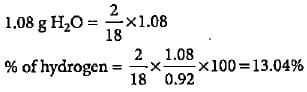Test: Quantitative Analysis (NCERT) - Question 2

### An organic compound gave 0.4655 g of CO2 on complete combustion. If the mass of the compound taken was 0.2115 g, what is the percentage of C in it?

Detailed Solution for Test: Quantitative Analysis (NCERT) - Question 2

Mass of CO2 formed = 0.4655 g
Mass of organic compound taken = 0.2115 g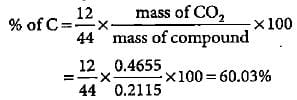Test: Quantitative Analysis (NCERT) - Question 3

### In Duma'smethod 0.52 g of an organic compound on combustion gave 68.6 mL N2 at 27oC and 76 mm pressure. What is the percentage of nitrogen in the compound?

Detailed Solution for Test: Quantitative Analysis (NCERT) - Question 3

V1 = 68.6 mL, P1 =. 756 mm, T1 = 300 K V2 = ?, P2 = 760 mm, T2 = 273 K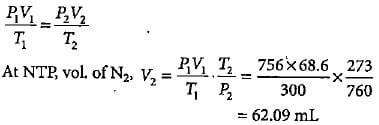Percentage of nitrogen in organic compound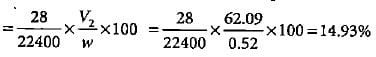Test: Quantitative Analysis (NCERT) - Question 4

In kjeldahl's method of estimation of nitrogen, nitrogen is quantitatively converted to ammonium sulphate. It is the treated with the standard solution of alkali. The nitrogen which is present is estimated as:

Detailed Solution for Test: Quantitative Analysis (NCERT) - Question 4

When treated with standard solution of alkali nitrogen is liberated as NH3 which is absorbed in H2SO4 to get percentage of nitrogen.

Test: Quantitative Analysis (NCERT) - Question 5

In Carius method of estimation of halogen, 0.15 g of an organic compound gave 0.12 g of AgBr. What is the percentage of bromine in the compound?

Detailed Solution for Test: Quantitative Analysis (NCERT) - Question 5

Molar mass of AgBr = 108 + 80 = 188 g mol-1
Percentage of Br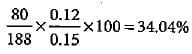Test: Quantitative Analysis (NCERT) - Question 6

2.18 g of an organic compound containing sulphur produces 1.02g of BaSO4. Thepercentage of sulphur in the compound is

Detailed Solution for Test: Quantitative Analysis (NCERT) - Question 6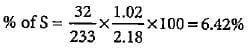Test: Quantitative Analysis (NCERT) - Question 7

1.6 g of an organic compound gave 2.6 g of magnesium pyrophosphate. The percentage of phosphorus in the compound is

Detailed Solution for Test: Quantitative Analysis (NCERT) - Question 7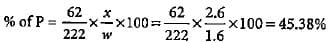Test: Quantitative Analysis (NCERT) - Question 8

Match the column I with column II in which formula for estimation of an element is given and mark the appropriate choice.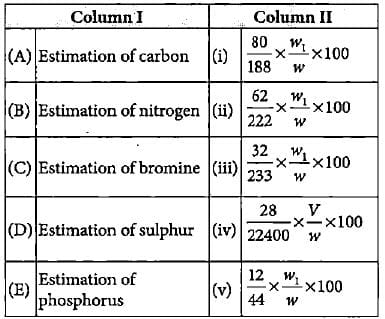Detailed Solution for Test: Quantitative Analysis (NCERT) - Question 8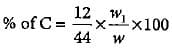(w1 = weight of CO2, w= weight of organic compound)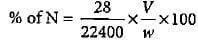(V = volume of N2 at STP, w = weight of organic compound)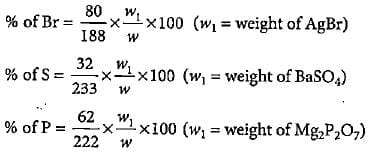Test: Quantitative Analysis (NCERT) - Question 9

The percentage of oxygen in heavy water is

Detailed Solution for Test: Quantitative Analysis (NCERT) - Question 9

In D2O percentage of deuterium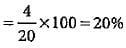% of oxygen - 100 - 20 = 80%

Test: Quantitative Analysis (NCERT) - Question 10

0.2 g of an organic compound contains C, H and O.On combustion, it yields 0.15 g CO2 and 0.12 g H2O.Ihe percentage of C, H and O respectively is

Detailed Solution for Test: Quantitative Analysis (NCERT) - Question 10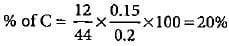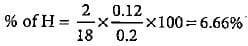% of O = 100 - (20 + 6.66) = 73.34%

## Chemistry Class 11

199 videos|330 docs|229 tests
Information about Test: Quantitative Analysis (NCERT) Page
In this test you can find the Exam questions for Test: Quantitative Analysis (NCERT) solved & explained in the simplest way possible. Besides giving Questions and answers for Test: Quantitative Analysis (NCERT), EduRev gives you an ample number of Online tests for practice

## Chemistry Class 11

199 videos|330 docs|229 tests

### How to Prepare for NEET

Read our guide to prepare for NEET which is created by Toppers & the best Teachers(Scan QR code)